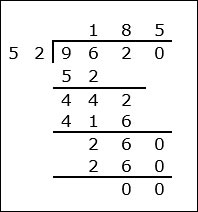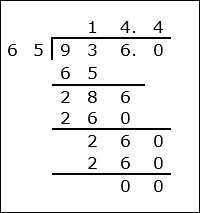# Division of a Decimal by a 2-digit Decimal

Division of a decimal by a decimal is similar to whole number division.

Rules for Decimal Division

• First we make both the decimals have the same number of decimal places if required.
• Then we divide them as if they were whole numbers ignoring the decimal points.
• The quotient so obtained is the required answer.

Divide 96.2 ÷ 0.52

### Solution

Step 1:

96.2 has one decimal place. 0.52 has two decimal places. So we make 96.2 have two decimal places by putting a zero to the right of 2 to make it 96.20.

Step 2:

Moving the decimal point to the right two places in both the numbers, it becomes a whole number division.

Now, 96.2 ÷ 0.52 = 96.20 ÷ 0.52 = 9620 ÷ 52 = 185Divide 9.36 ÷ 0.65

### Solution

Step 1:

0.65 has two decimal places. 9.36 has two decimal places.

Step 2:

Now, 9.36 ÷ 0.65 = 936 ÷ 65 = 14.4